Courses

# Value Based Questions- Areas Related to Circles Class 10 Notes | EduRev

## Class 10 : Value Based Questions- Areas Related to Circles Class 10 Notes | EduRev

The document Value Based Questions- Areas Related to Circles Class 10 Notes | EduRev is a part of the Class 10 Course Class 10 Mathematics by VP Classes.
All you need of Class 10 at this link: Class 10

Q1. Shankar wants a lawn to be developed in a corner of his square plot. He gave following three options to the lawn-developer: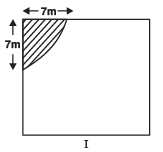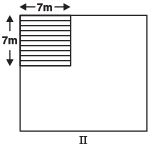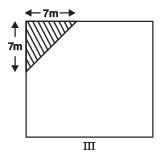The lawn developing rate is Rs 150 per sq. m and the lawn under option-III was developed, however, Shankar paid for the option having largest area. The lawn-developer realized the mistake and refunded the balance back to Shankar.
(a) Find the area in each of the above three options.
(b) What amount was refunded back to Shankar by the lawn-developer?
(c) Which mathematical concept is used in above problem?
(d) Refunding the difference, which value is depicted by the lawn-developer?

Sol. (a) Area of lawn in option-I = 1/4 (πr2)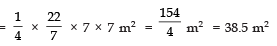Area of lawn in option-II = 7 × 7 m2 = 49 m2

Area of lawn in option-III =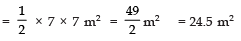(b) ∵ Largest area of the lawn = 49 m2
Cost of lawn-developing in option-II = Rs 150 × 49 = Rs 7350
∵ Area of lawn in option-III = 24.5 m2
∴ Cost of lawn-developing in option-III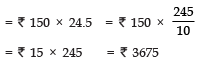⇒ Amount refunded back by the lawn-developer = Rs 7350 – Rs 3675 = Rs 3675
(c) Areas related to plane surfaces.
(d) Honesty

Q2. Shivram has a piece of land in the form of sector OBPQ adjoining to a temple. He donates a part of it to the temple such that a square plot OABC is left with him.
If OA = 20 m. Then,
(a) Find the area of the shaded region (donated to the temple). [use π = 3.14]
(b) Which mathematical concept is used in above problem?
(c) By donating land to a temple by Shivram, which value is depicted?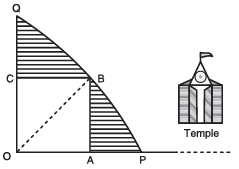Sol. (a) ∵ OABC is a square and OA = 20 m
∴ Diagonal OB of the square OABC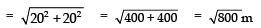⇒ Radius of the quadrant OPBQ =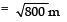⇒ Area of the quadrant OPBQ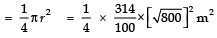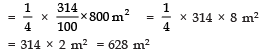Also, area of square OABC = 20 × 20 m2 = 400 m2
∴ Area of the shaded region = [Area of the quadrant OPBQ] – [Area of the square OABC]
= 628 m2 – 400 m2 = 228 m2

Thus, area of the land donated to the temple = 228 m2
(b) Related to circles
(c) Charity.

Q3. Rajat has a piece of land in the shape of a rectangle with two semicircles on its smaller sides, as diameters, added to its outside. The sides of the rectangle are 36 m and 21 m. He lets out the two semicircular parts at the rate of Rs 100 per sq. metre and donates the proceeds to an orphanage.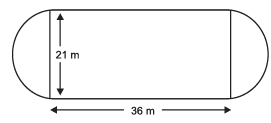(a) Find the total area of his land. [use π = 22/7]
(b) What amount does he donate to orphanage?
(c) Which mathematical concept is used in the above problem?
(d) By donating the rent proceeds to an orphanage which value is depicted by Rajat?

Sol. (a) Area of the rectangular part = 36 × 24.5 m2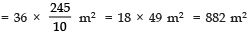Area of both the semicircular parts =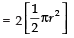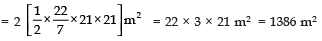∴ Area of Rajat’s total land = 882 m2 + 1386 m2 = 2268 m2
(b) ∴ Rent rate = Rs 100 per m2
∴ Total rent = Rs 1386 × 100 = Rs 1,38,600
⇒ Amount donated to orphanage by Rajat = Rs 1,38,600
(c) Areas related to circles

Q4. Rajesh has a circular plot of radius 105m. He donates a 7m wide track along its boundary for community-track.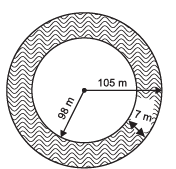(a) Find the area of the track. [use π = 22/7]
(b) Which mathematical concept is used in the above problem?
(c) By donating a community-track, which value is depicted by Rajesh?

Sol. (a) Radius of the total circular plot = 105 m
∴ Area of the total circular plot = π (105)2 sq. m
∴ Width of the track = 7 m
∴ Radius of the circular plot excluding the track
= 105 m – 7 m = 98 m
⇒ Area of the inner circular plot = π (98)2 sq. m.
Now, area of the track = [Area of total circular plot] – [Area of inner circular plot]
= π (105)2 – π (98)2 sq. m
= π [1052 – 982] sq. m
= π [(105 – 98) (105 + 98)] sq. m
[using a2 – b2 = (a–b) (a+b)]

= π [7 × 203] sq. m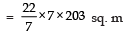= 22 × 203 sq. m
= 4466 sq. m

(b) Areas related to circles
(c) Community service

Offer running on EduRev: Apply code STAYHOME200 to get INR 200 off on our premium plan EduRev Infinity!

132 docs

,

,

,

,

,

,

,

,

,

,

,

,

,

,

,

,

,

,

,

,

,

;